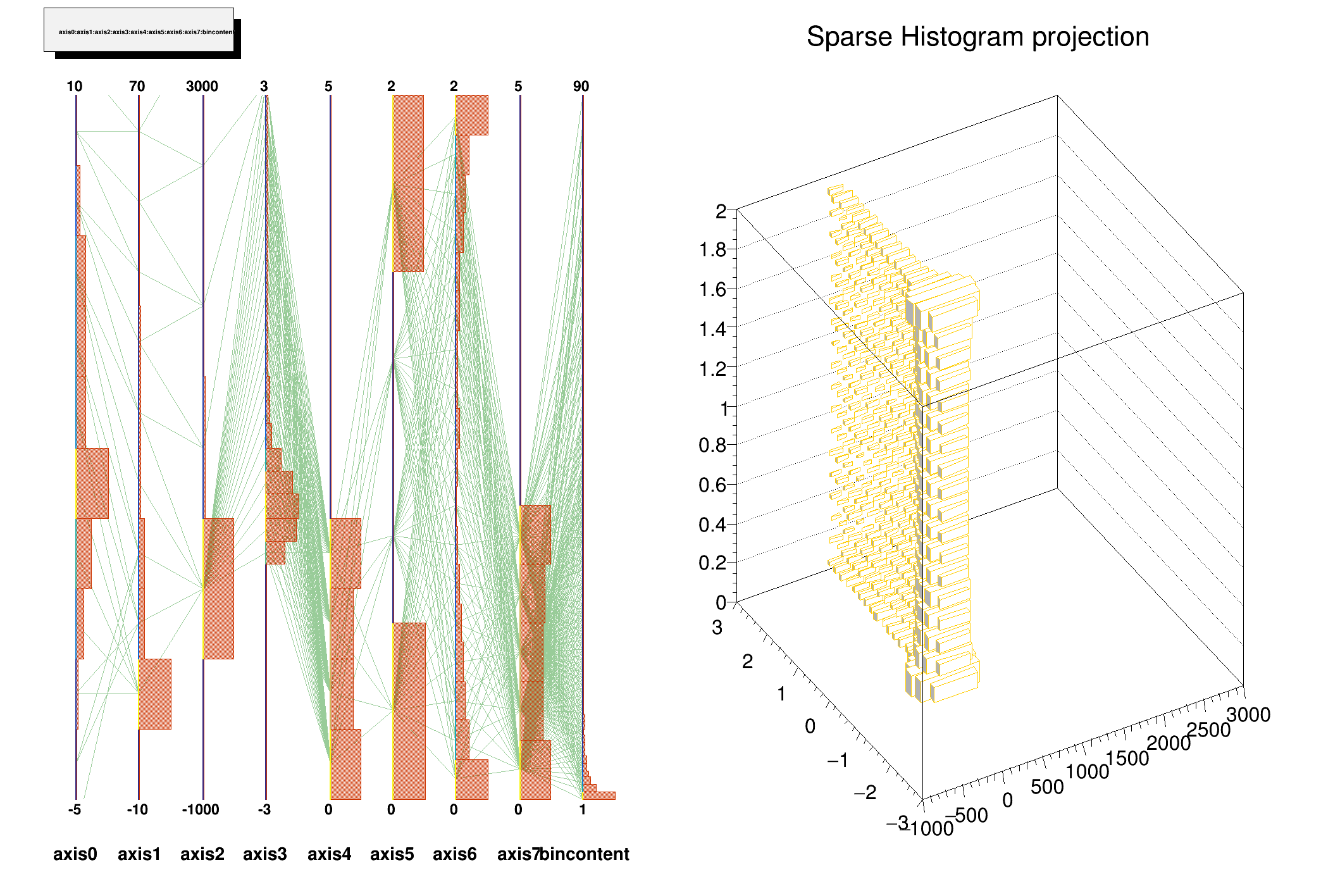ROOT   Reference Guide
Searching...
No Matches
drawsparse.C File Reference

## Detailed DescriptionConvert a THnSparse to a TTree using efficient iteration through the THnSparse and draw a THnSparse using TParallelCoord.

The plot will contain one line for each filled bin, with the bin's coordinates on each axis, and the bin's content on the rightmost axis.

Run as

.L \$ROOTSYS/tutorials/tree/drawsparse.C+
Definition tree.py:1#include "TParallelCoord.h"
#include "TROOT.h"
#include "TTree.h"
#include "TLeaf.h"
#include "THnSparse.h"
#include "TAxis.h"
#include "TCanvas.h"
#include "TRandom.h"
#include "TFile.h"
#include "TH3.h"
TTree* toTree(THnSparse* h)
{
// Creates a TTree and fills it with the coordinates of all
// filled bins. The tree will have one branch for each dimension,
// and one for the bin content.
Int_t dim = h->GetNdimensions();
TString name(h->GetName()); name += "_tree";
TString title(h->GetTitle()); title += " tree";
TTree* tree = new TTree(name, title);
Double_t* x = new Double_t[dim + 1];
memset(x, 0, sizeof(Double_t) * (dim + 1));
TString branchname;
for (Int_t d = 0; d < dim; ++d) {
if (branchname.Length())
branchname += ":";
TAxis* axis = h->GetAxis(d);
branchname += axis->GetName();
branchname += "/D";
}
tree->Branch("coord", x, branchname);
tree->Branch("bincontent", &x[dim], "bincontent/D");
Int_t *bins = new Int_t[dim];
for (Long64_t i = 0; i < h->GetNbins(); ++i) {
x[dim] = h->GetBinContent(i, bins);
for (Int_t d = 0; d < dim; ++d) {
x[d] = h->GetAxis(d)->GetBinCenter(bins[d]);
}
tree->Fill();
}
delete [] bins;
//delete [] x;
return tree;
}
void drawsparse_draw(THnSparse* h)
{
// Draw a THnSparse using TParallelCoord, creating a temporary TTree.
TTree* tree = toTree(h);
TString whatToDraw;
TIter iLeaf(tree->GetListOfLeaves());
const TLeaf* leaf = 0;
while ((leaf = (const TLeaf*)iLeaf())) {
if (whatToDraw.Length())
whatToDraw += ":";
whatToDraw += leaf->GetName();
}
tree->Draw(whatToDraw, "", "para");
TIter iVar(parallelCoord->GetVarList());
for (Int_t d = 0;(var = (TParallelCoordVar*) iVar()) && d < h->GetNdimensions(); ++d) {
TAxis* axis = h->GetAxis(d);
var->SetCurrentLimits(axis->GetXmin(), axis->GetXmax());
var->SetTitle(axis->GetTitle());
}
var->SetTitle("bin content");
}
void drawsparse()
{
// create a THnSparse and draw it.
const Int_t ndims = 8;
Int_t bins[ndims] = {10, 10, 5, 30, 10, 4, 18, 12};
Double_t xmin[ndims] = {-5., -10., -1000., -3., 0., 0., 0., 0.};
Double_t xmax[ndims] = {10., 70., 3000., 3., 5., 2., 2., 5.};
THnSparse* hs = new THnSparseD("hs", "Sparse Histogram", ndims, bins, xmin, xmax);
// fill it
Double_t x[ndims];
for (Long_t i = 0; i < 100000; ++i) {
for (Int_t d = 0; d < ndims; ++d) {
switch (d) {
case 0: x[d] = gRandom->Gaus()*2 + 3.; break;
case 1:
case 2:
case 3: x[d] = (x[d-1]*x[d-1] - 1.5)/1.5 + (0.5*gRandom->Rndm()); break;
default: x[d] = sin(gRandom->Gaus()*i/1000.) + 1.;
}
}
hs->Fill(x);
}
TFile* f = new TFile("drawsparse.root","RECREATE");
TCanvas* canv = new TCanvas("hDrawSparse", "Drawing a sparse hist");
canv->Divide(2);
// draw it
canv->cd(1);
drawsparse_draw(hs);
// project it
canv->cd(2);
TH3D* h3proj = hs->Projection(2, 3, 6);
h3proj->SetDirectory(0);
h3proj->Draw("lego1");
// save everything to a file
canv->Write();
hs->Write();
h3proj->Write();
delete f;
}
#define d(i)
Definition RSha256.hxx:102
#define f(i)
Definition RSha256.hxx:104
#define h(i)
Definition RSha256.hxx:106
int Int_t
Definition RtypesCore.h:45
long Long_t
Definition RtypesCore.h:54
double Double_t
Definition RtypesCore.h:59
long long Long64_t
Definition RtypesCore.h:73
@ kOrange
Definition Rtypes.h:67
char name
Definition TGX11.cxx:110
float xmin
float xmax
THnSparseT< TArrayD > THnSparseD
Definition THnSparse.h:219
double sin(double)
R__EXTERN TRandom * gRandom
Definition TRandom.h:62
virtual void SetLineColor(Color_t lcolor)
Set the line color.
Definition TAttLine.h:40
Class to manage histogram axis.
Definition TAxis.h:30
Double_t GetXmax() const
Definition TAxis.h:134
Double_t GetXmin() const
Definition TAxis.h:133
Int_t GetNbins() const
Definition TAxis.h:121
const char * GetTitle() const
Returns title of object.
Definition TAxis.h:129
The Canvas class.
Definition TCanvas.h:23
Definition TCanvas.cxx:708
A ROOT file is a suite of consecutive data records (TKey instances) with a well defined format.
Definition TFile.h:54
virtual void SetDirectory(TDirectory *dir)
By default when an histogram is created, it is added to the list of histogram objects in the current ...
Definition TH1.cxx:8777
virtual void Draw(Option_t *option="")
Draw this histogram with options.
Definition TH1.cxx:3073
3-D histogram with a double per channel (see TH1 documentation)}
Definition TH3.h:305
Long64_t Fill(const Double_t *x, Double_t w=1.)
Definition THnBase.h:144
Efficient multidimensional histogram.
Definition THnSparse.h:36
TH1D * Projection(Int_t xDim, Option_t *option="") const
Forwards to THnBase::Projection().
Definition THnSparse.h:133
A TLeaf describes individual elements of a TBranch See TBranch structure in TTree.
Definition TLeaf.h:57
virtual const char * GetName() const
Returns name of object.
Definition TNamed.h:47
virtual Int_t Write(const char *name=0, Int_t option=0, Int_t bufsize=0)
Write this object to the current directory.
Definition TObject.cxx:798
virtual TObject * FindObject(const char *name) const
Must be redefined in derived classes.
Definition TObject.cxx:323
void Divide(Int_t nx=1, Int_t ny=1, Float_t xmargin=0.01, Float_t ymargin=0.01, Int_t color=0) override
TParallelCoord axes.
void SetTitle(const char *)
Set the title of the TNamed.
void SetHistogramBinning(Int_t n=100)
Set the histogram binning.
void SetCurrentLimits(Double_t min, Double_t max)
Set the limits within which one the entries must be painted.
Parallel Coordinates class.
TList * GetVarList()
virtual Double_t Gaus(Double_t mean=0, Double_t sigma=1)
Samples a random number from the standard Normal (Gaussian) Distribution with the given mean and sigm...
Definition TRandom.cxx:274
virtual Double_t Rndm()
Machine independent random number generator.
Definition TRandom.cxx:552
Basic string class.
Definition TString.h:136
Ssiz_t Length() const
Definition TString.h:410
A TTree represents a columnar dataset.
Definition TTree.h:79
Double_t x[n]
Definition legend1.C:17

Definition in file drawsparse.C.